﻿ Vienna Center for Partial Differential Equations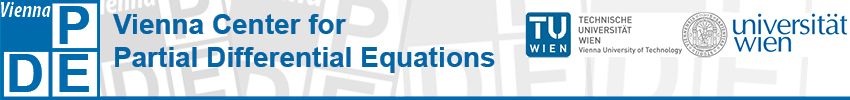# MembersAnton Arnold Anton Arnold is Professor at the Institute for Analysis and Scientific Computing, Vienna University of Technology. His research is concerned with the analysis and numerical analysis of partial differential equations. In particular he is interested in the large-time behavior of parabolic and kinetic equations, open quantum systems as well as polymeric fluid flow models. Other topics of his research include open boundary conditions for Schrödinger-type equations as well as numerically efficient WKB-schemes for highly oscillatory problems. anton.arnold@tuwien.ac.at Webpage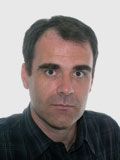Adrian Constantin Adrian Constantin is Professor of Mathematics at the University of Vienna and is the current holder of the Chair in Analysis at King's College London. His main area of research is the theory of nonlinear partial differential equations and its applications. While he is foremost an analyst, his work joins together pure and applied mathematics. He worked on integrable systems and soliton theory, dynamical systems and free boundary problems in fluid mechanics. Another area of his research is geometric mechanics. He also wrote several papers devoted to aspects relevant to tsunami waves. adrian.constantin@univie.ac.at Webpage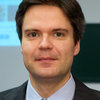Clemens Heitzinger Clemens Heitzinger is Professor at the Institute for Analysis and Scientific Computing of Vienna University of Technology. His research is concerned with the analysis and numerical analysis of deterministic and stochastic partial differential equations. In particular, he is interested in multiscale problems and homogenization as well as uncertainty quantification.  He also develops simulation capabilities to provide the quantitative understanding of new and emerging technologies; an example are his results for new nanowire sensors. clemens.heitzinger@tuwien.ac.at Webpage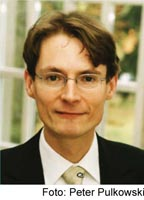Ansgar Jüngel Ansgar Jüngel is Professor at the Institute for Analysis and Scientific Computing of Vienna University of Technology and speaker of the Doctoral School Dissipation and Dispersion in Nonlinear PDEs. His research is concerned with the mathematical modeling, analysis, and numerical approximation of nonlinear PDEs. One main topic is the derivation and analysis of semiconductor models and the numerical simulation of semiconductor devices. He is also involved in the development of entropy dissipation methods for nonlinear evolution equations and cross-diffusion systems arising in physics and biology. ansgar.juengel@tuwien.ac.at Webpage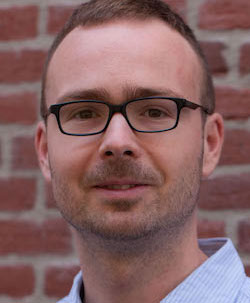Jan Maas Jan Maas is Assistant Professor of Stochastic Analysis at The Institute of Science and Technology Austria. His research interests are at the interface of mathematical analysis and probability theory. Recent work is concerned with the theory of optimal transport and its applications to stochastic processes, chemical reaction networks and quantum Markov processes. Another main research area is the analysis of stochastic partial differential equations, with a particular focus on the approximation theory for highly irregular equations. jan.maas@ist.ac.at WebpagePeter Markowich Peter Markowich is Professor of Mathematics at the University of Vienna. He has also affiliations with the University of Cambridge (United Kingdom) and the King Abdullah University of Science and Technology (KAUST, Saudi-Arabia). His research field is the mathematical and numerical analysis of partial differential equations and their application in physics, biology, and engineering. In particular, he is interested in classical and quantum mechanical kinetic theory, analytical and numerical problems occuring in highly oscillatory differential equations, and Wigner transforms. peter.markowich@univie.ac.at Webpage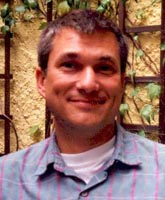Norbert Mauser Norbert J. Mauser is Professor of Mathematics at the University of Vienna, Director of the Wolfgang Pauli Institute (WPI) and the Institut CNRS Pauli, UMI 2842 du CNRS. His research field is modeling, (asymptotic) analysis, and numerical simulation of time dependent nonlinear PDEs. In particular, he is working on generalized nonlinear Schrödinger equations in quantum physics, e.g. the Gross-Pitaevskii equation modeling Bose-Einstein condensates or Dirac and Pauli equation, MCTDHF and TDDFT modeling electron systems. mauser@courant.nyu.edu Webpage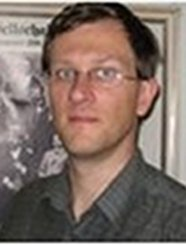Jens Markus Melenk Jens Markus Melenk is Professor of Computational Mathematics at the Vienna University of Technology and head of the Institute for Analysis and Scientific Computing. Melenk's research centers around high order discretizations of (elliptic) PDEs such as stability and convergence analysis (including regularity theory for elliptic problems), adaptivity (encompassing error estimation, convergence optimality), fast implementation, and solver issues. His interests associated with the DK are finite element and boundary element methods for wave propagation problems. melenk@tuwien.ac.at Webpage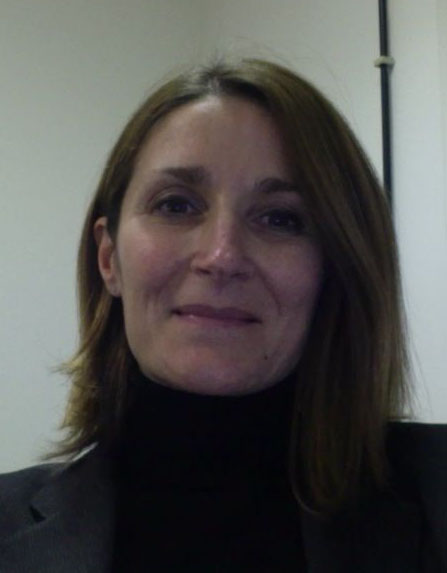Ilaria PerugiaIlaria Perugia is Professor of Numerics of Partial Differential Equations at the Faculty of Mathematics, University of Vienna. Her research is concerned with finite element methods for the numerical approximation of PDEs. Her interests mainly focus on the design and the analysis of non standard finite element methods, such as discontinuous Galerkin methods (for elliptic source and eigenvalue problems, reaction-diffusion problems) and finite elements with operator-adapted basis functions (for wave propagation problems). ilaria.perugia@univie.ac.at WebpageDirk Praetorius Dirk Praetorius is Professor at the Institute for Analysis and Scientific Computing of Vienna University of Technology. His research deals with certain aspects of numerical analysis for partial differential equations, in particular, the finite element method and the boundary element method. One focus lies on the development of effective a posteriori error estimators and the convergence and quasi-optimality analysis of the corresponding adaptive mesh-refining algorithms. A second focus is on effective numerical methods for computational micromagnetics. dirk.praetorius@tuwien.ac.at Webpage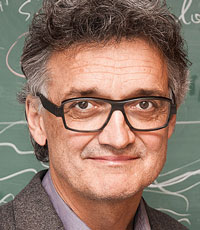Walter Schachermayer Walter Schachermayer is Professor at the Faculty of Mathematics of the University of Vienna. His main research interest lies in Mathematical Finance, Stochastic Analysis, Functional Analysis and Probability Theory. He is well-known for his work on the fundamental theorem of asset pricing, portfolio optimization and models under transaction costs. He is also interested in problems of optimal transport theory and their relations to martingale theory and model-free mathematical finance. Photo: Suchart Wannaset. Walter.Schachermayer@univie.ac.at WebpageChristian Schmeiser Christian Schmeiser is Professor at the Faculty of Mathematics, University of Vienna. His research has been concerned with modeling, analysis, and simulation of differential equations and stochastic processes, with applications in physics, engineering, and biology. Some of this work contributed to kinetic transport theory, in particular to the rigorous derivation of macroscopic limits. One of his main interests is in Mathematical Cell Biology, where he contributed to the theory of chemotaxis and, in an interdisiplinary cooperation, to the modeling of cytoskeleton dynamics. Christian.Schmeiser@univie.ac.at WebpageJoachim Schöberl Joachim Schöberl is Professor at the Institute for Analysis and Scientific Computing of Vienna University of Technology. His research focuses on the numerical approximation of partial differential equations with the finite element method. He is interested in the design and analysis of methods such as e.g. high order, mixed and discontinuous Galerkin methods, robust multi-level and domain decomposition preconditioning, or a posteriori error estimators, as well in their application to engineering challenges in electromagnetics, solid-mechanics and fluid dynamics. joachim.schoeberl@tuwien.ac.at WebpageUlisse Stefanelli Ulisse Stefanelli holds the Chair of Applied Mathematics and Modeling at the University of Vienna. His research focuses on partial differential equations and calculus of variations. He is interested in abstract and applied problems from a number of different perspectives including modeling, analysis, asymptotics, and numerical approximation. Main topic of his research is the analysis of variational structures, especially in the nonlinear evolutive context. In particular, he has been working in Materials Science, phase transitions, and Thermomechanics. ulisse.stefanelli@univie.ac.at Webpage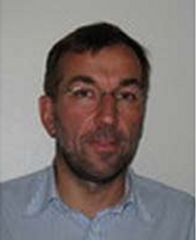Peter Szmolyan Peter Szmolyan is Professor at the Institute for Analysis and Scientific Computing of Vienna University of Technology. His research is concerned with the dynamics of multi-scale problems, geometric approaches to singular limits and asymptotic analysis, local and global bifurcations, as well as the dynamics of nonlinear waves and related patterns. Significant parts of his research are driven by applications, e.g. from biology, physics, and engineering. He has made important contributions to the field of geometric singular perturbation theory and to the area of viscous conservation laws. szmolyan@tuwien.ac.at WebpageGerald Teschl Gerald Teschl is Professor at the Faculty of Mathematics, University of Vienna. His research focuses on direct and inverse spectral theory for differential and difference operators in connection with completely integrable nonlinear wave equations. Main topics of his research are for example inverse scattering transform for integrable wave equations or long-time asymptotics using the nonlinear steepest descent analysis. A recent direction is the long-time asymptotics of the dispersionless Camassa-Holm equations. Gerald.Teschl@univie.ac.at WebpageMarie-Therese Wolfram Marie-Therese Wolfram leads the New Frontiers Group on Multiscale modelling and simulation of crowded transport in the life and social sciences at the Radon Institute for Computational and Applied Mathematics, Austrian Academy of Sciences. A common theme of her research are transport phenomena, ranging from pedestrian dynamics to ion transport or price formation models. She tries to understand the complex dynamics of these processes by deriving consistent mathematical models and analyzing them using PDE techniques as well as numerical simulations. mt.wolfram@ricam.oeaw.ac.at Webpage